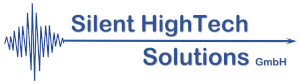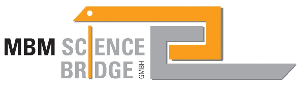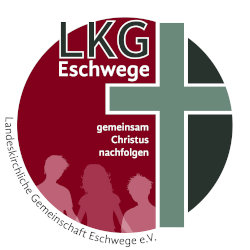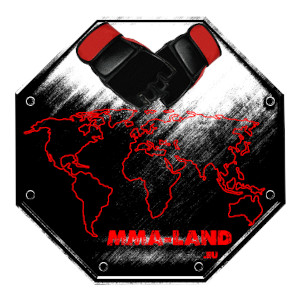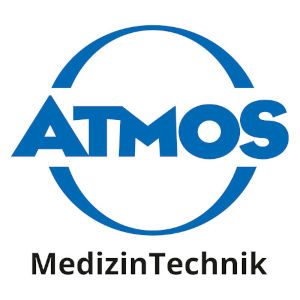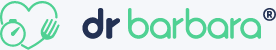Digital Tbucket Tank (DTT)

# Mathematics for computer scientists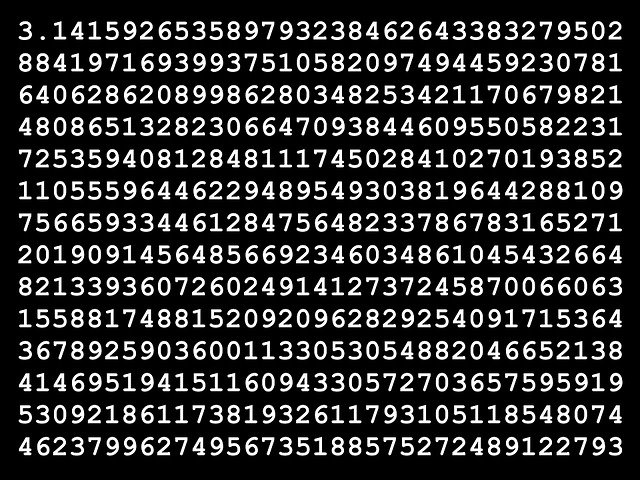Computer science is the study of computation, automation, and information. Computer science encompasses theoretical disciplines (such as algorithms, computational theory, and information theory) and practical disciplines (including the design and implementation of hardware and software). Computer science is generally viewed as an academic research field considered and differentiated from computer programming.

Algorithms and data structures are of central importance for computer science. Computational theory deals with abstract computational models and general classes of problems that can be solved with them. The fields of cryptography and computer security deal with the study of means of secure communication and the prevention of security vulnerabilities. Computer graphics and computer geometry deal with the creation of images. The programming language theory deals with approaches to the description of computing processes, and the database theory with the management of databases.

Fokus

Mathematical basics

Mengen

propositional logic

number systems

Illustrations

Basic algebraic structures

Prime numbers

Modular arithmetic

Application in cryptography

Introduction to Matrices

Invert matrices

Systems of linear equations

Introduction to graphs

problem of shortest paths

The Königsberg bridge problem

A city tour where every city is visited once

Trees and Knot Theory

### Customers and Partners